You are currently offline. Some features of the site may not work correctly.

# System of linear equations

Known as: Homogeneous system, Linear Equation System, Vector equation
In mathematics, a system of linear equations (or linear system) is a collection of two or more linear equations involving the same set of variables… Expand
Wikipedia

## Papers overview

Semantic Scholar uses AI to extract papers important to this topic.
Review
2016
Review
2016
Machine learning techniques are applied for solving a large variety of practical problems. The tasks attacked by machine learning… Expand
Is this relevant?
Highly Cited
2012
Highly Cited
2012
• IEEE Transactions on Information Theory
• 2012
• Corpus ID: 7923170
Finding the sparsest solution to underdetermined systems of linear equations y = Φx is NP-hard in general. We show here that for… Expand
•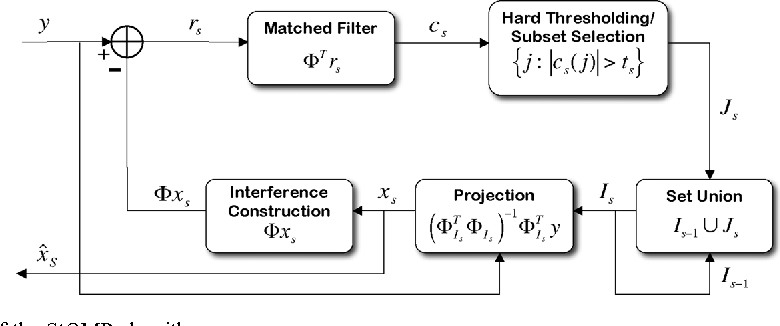•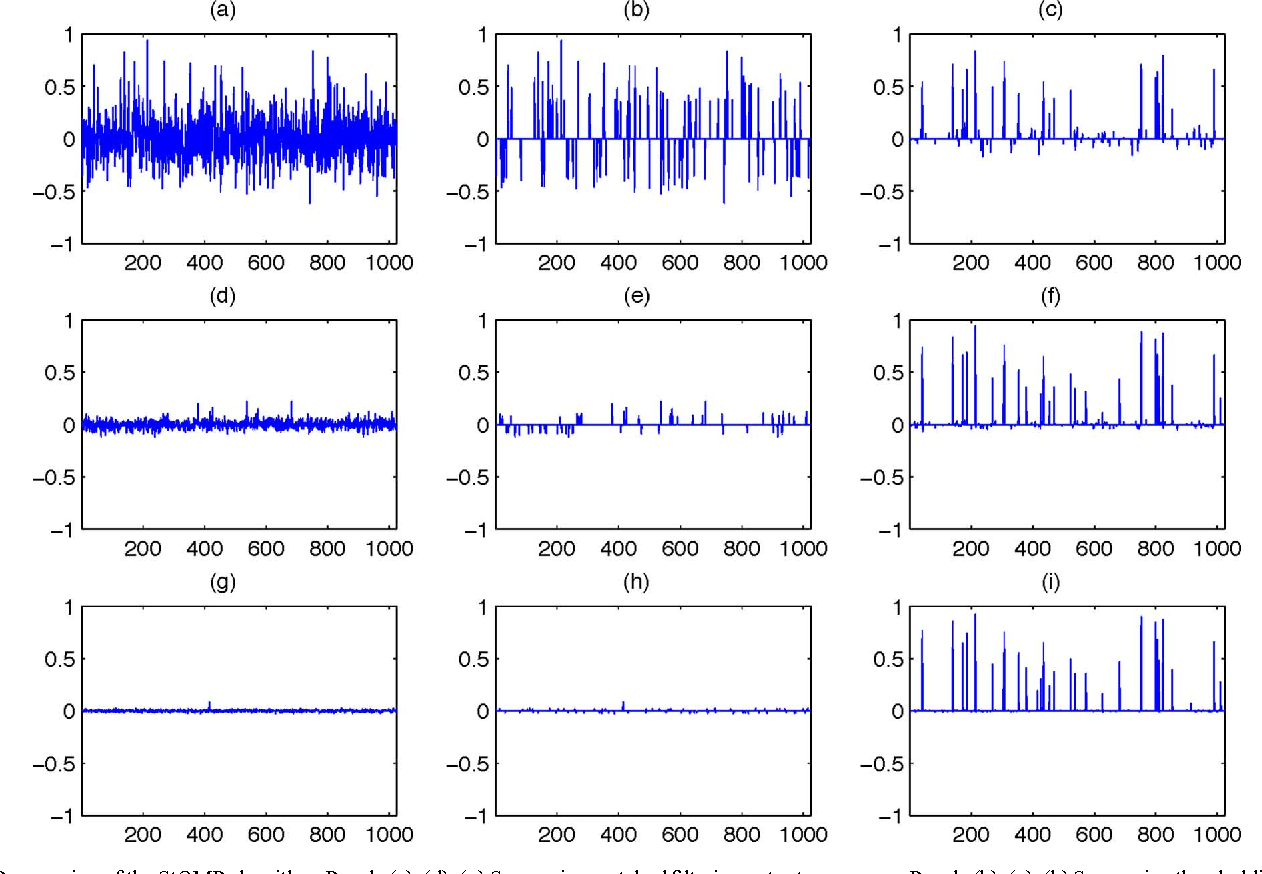•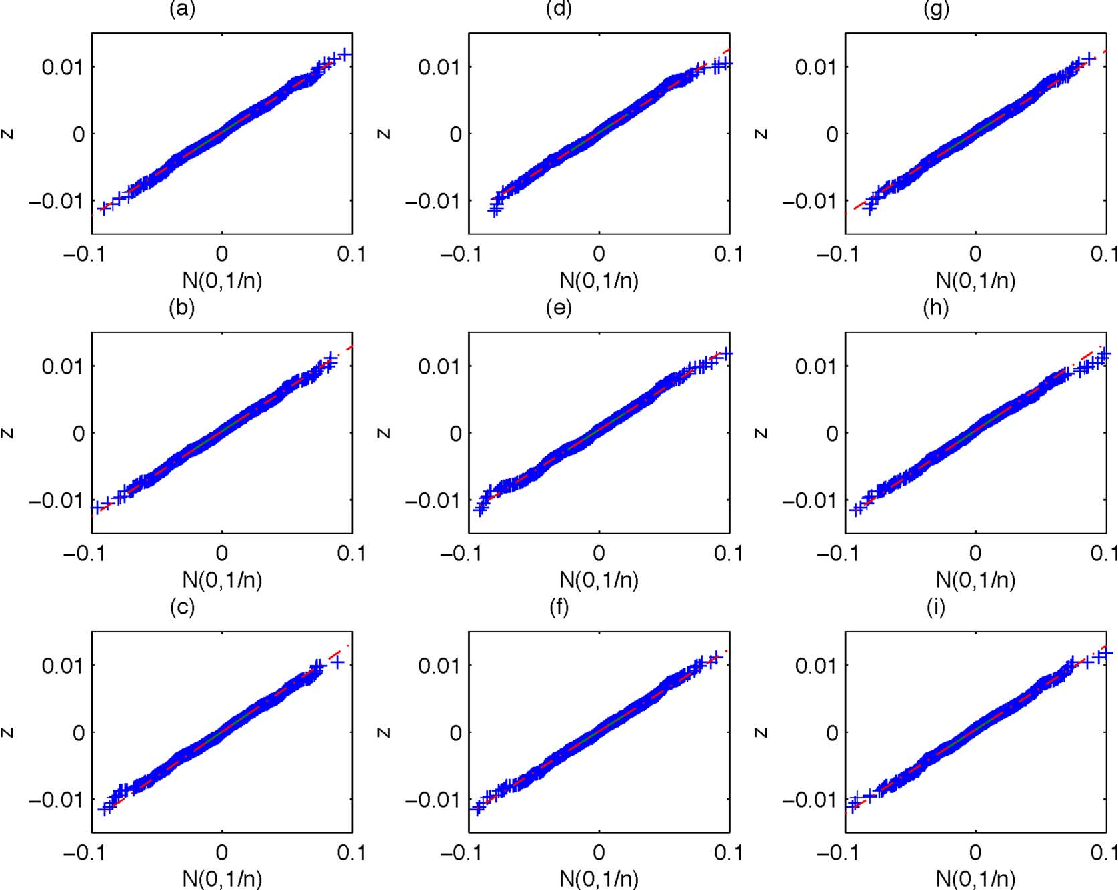•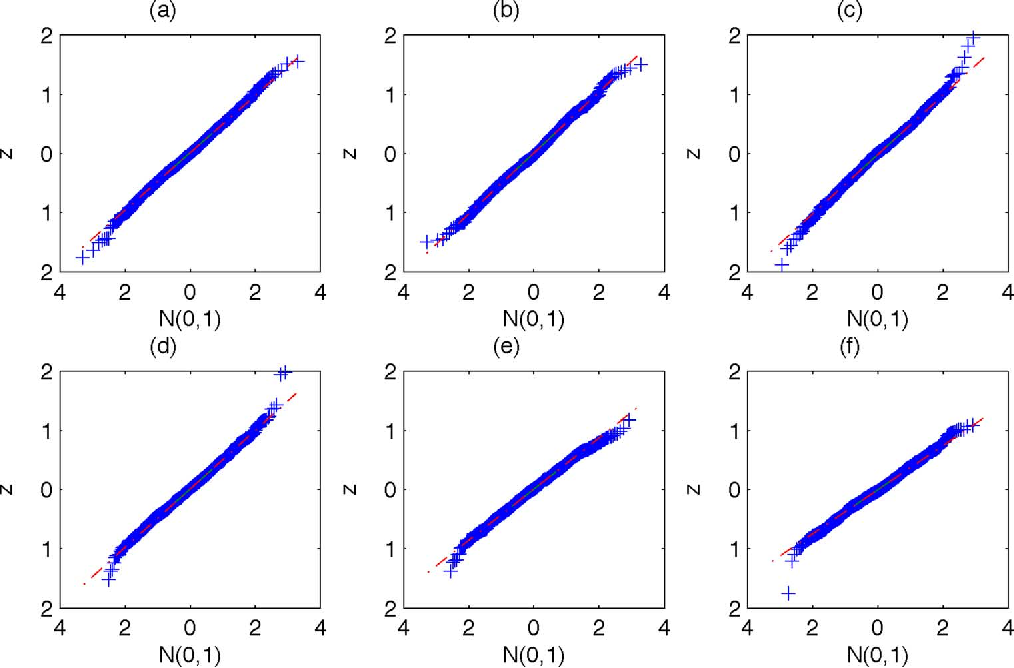•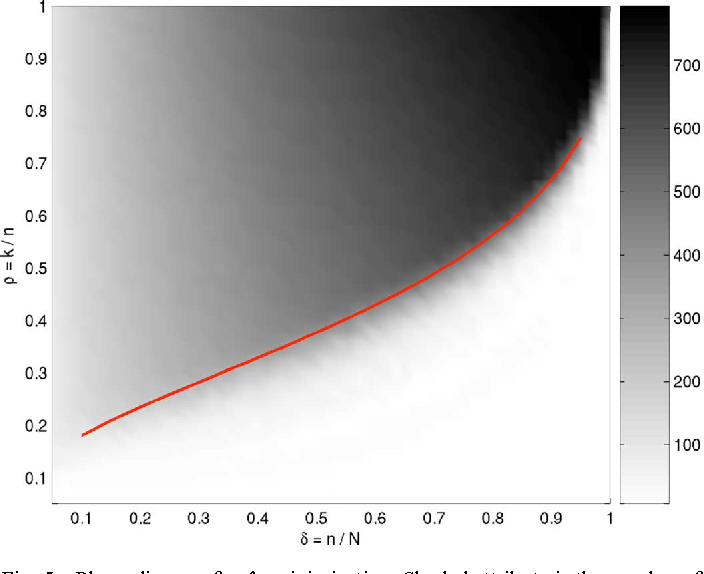Is this relevant?
Highly Cited
2006
Highly Cited
2006
We consider linear equations y = Φx where y is a given vector in ℝn and Φ is a given n × m matrix with n 0 so that for large n… Expand
Is this relevant?
Highly Cited
2006
Highly Cited
2006
Finding the sparsest solution to underdetermined systems of linear equations y = Φx is NP-hard in general. We show here that for… Expand
•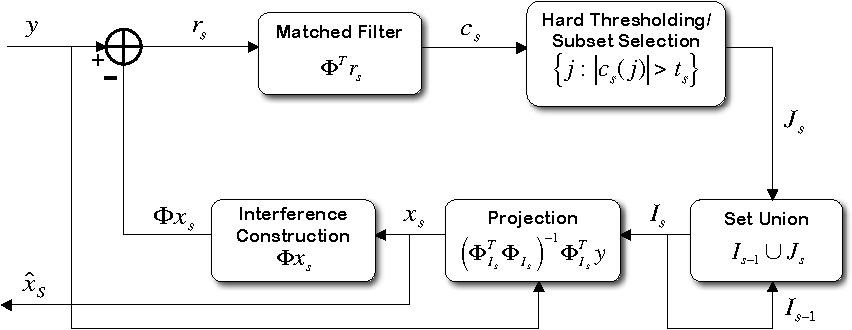•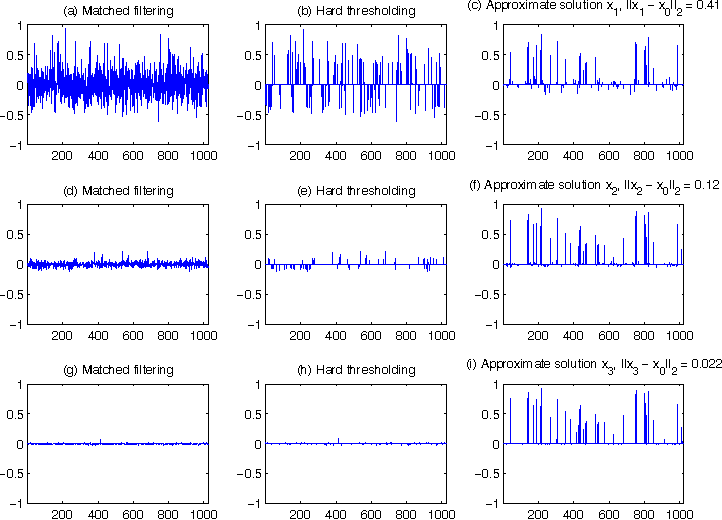•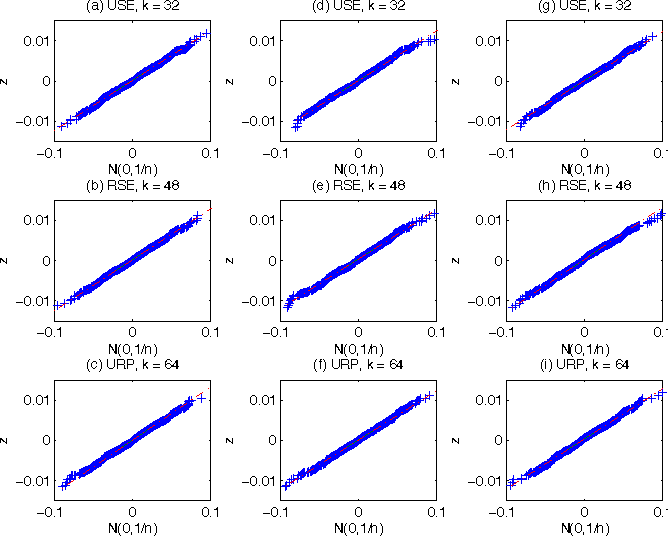•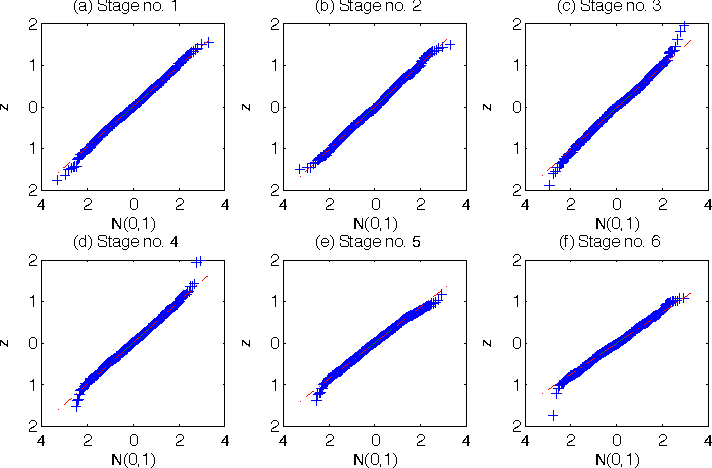•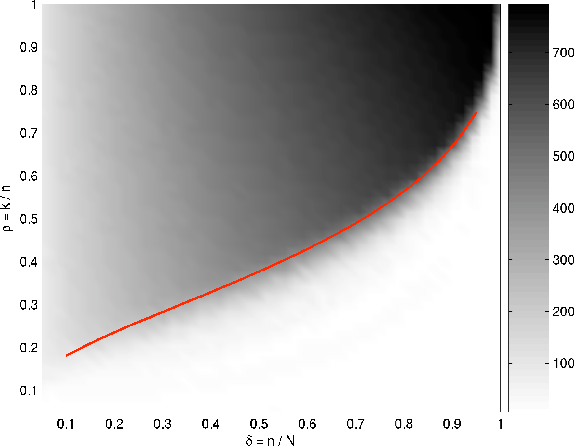Is this relevant?
Highly Cited
2004
Highly Cited
2004
• Future Gener. Comput. Syst.
• 2004
• Corpus ID: 35511249
Supernode partitioning for unsymmetric matrices together with complete block diagonal supernode pivoting and asynchronous… Expand
Is this relevant?
Highly Cited
1994
Highly Cited
1994
• R. Barrett
• Software, environments, tools
• 1994
• Corpus ID: 15815197
In this book, which focuses on the use of iterative methods for solving large sparse systems of linear equations, templates are… Expand
Is this relevant?
Highly Cited
1986
Highly Cited
1986
A "coordinate recurrence" method for solving sparse systems of linear equations over finite fields is described. The algorithms… Expand
Is this relevant?
Highly Cited
1983
Highly Cited
1983
• 1983
• Corpus ID: 2456661
We consider a class of iterative algorithms for solving systems of linear equations where the coefficient matrix is nonsymmetric… Expand
Is this relevant?
Highly Cited
1965
Highly Cited
1965
• 1965
• Corpus ID: 55364462
Abstract : From a theory of Hohenberg and Kohn, approximation methods for treating an inhomogeneous system of interacting… Expand
Is this relevant?
Highly Cited
1952
Highly Cited
1952
In an earlier publication  a method was described which generated the eigenvalues and eigenvectors of a matrix by a… Expand
•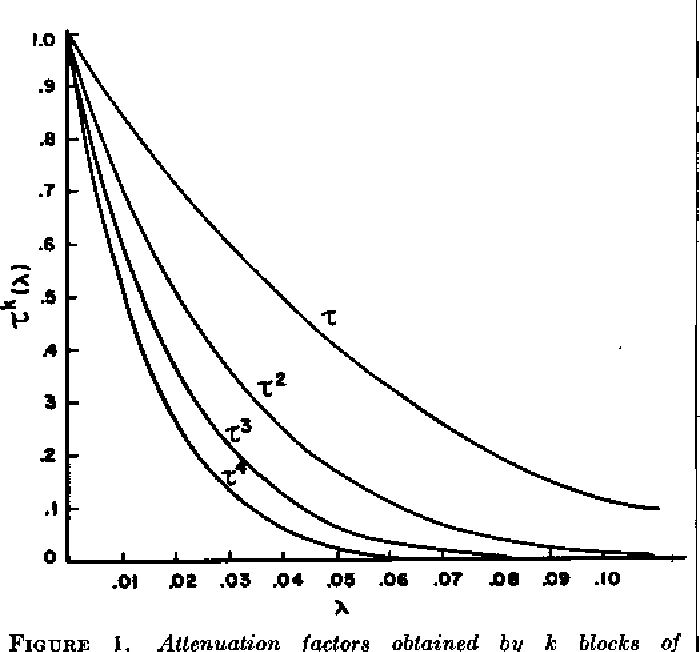•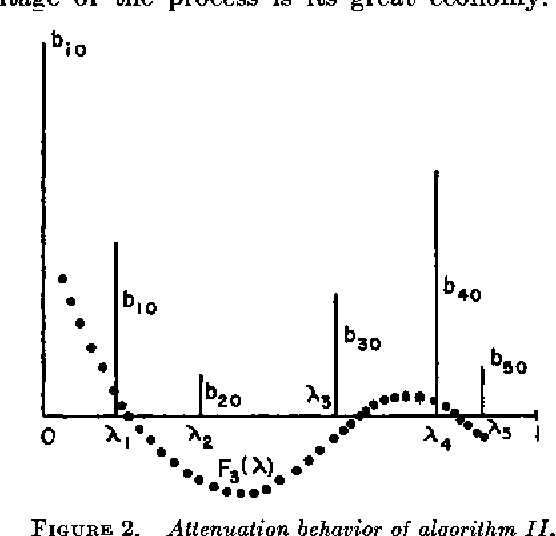Is this relevant?